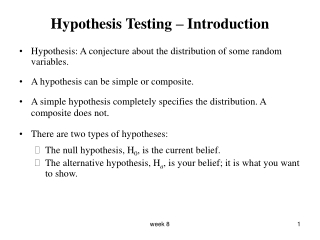DownloadDownload PresentationHypothesis Testing – Introduction

# Hypothesis Testing – Introduction

Télécharger la présentation## Hypothesis Testing – Introduction

- - - - - - - - - - - - - - - - - - - - - - - - - - - E N D - - - - - - - - - - - - - - - - - - - - - - - - - - -
##### Presentation Transcript

1. Hypothesis Testing – Introduction • Hypothesis: A conjecture about the distribution of some random variables. • A hypothesis can be simple or composite. • A simple hypothesis completely specifies the distribution. A composite does not. • There are two types of hypotheses: • The null hypothesis, H0, is the current belief. • The alternative hypothesis, Ha, is your belief; it is what you want to show. week 8

2. Testing Process • Hypothesis testing is a proof by contradiction. • The testing process has four steps: • Step 1: Assume H0 is true. • Step 2: Use statistical theory to make a statistic (function of the data) that includes H0. This statistic is called the test statistic. • Step 3: Find the probability that the test statistic would take a value as extreme or more extreme than that actually observed. Think of this as: probability of getting our sample assuming H0 is true. • Step 4: If the probability we calculated in step 3 is high it means that the sample is likely under H0 and so we have no evidence against H0. If the probability is low it means that the sample is unlikely under H0. This in turn means one of two things; either H0 is false or we are unlucky and H0 is true. week 8

3. Example week 8

4. Graphical Representation • Let Sn be the set of all possible samples of size n from the population we are sampling from. • Let C be the set of all samples for which we reject H0. It is called the critical region. • is the set of all samples for which we fail to reject H0. It is called the acceptance region. week 8

5. Example week 8

6. Decision Errors • When we perform a statistical test we hope that our decision will be correct, but sometimes it will be wrong. There are two possible errors that can be made in hypothesis test. • The error made by rejecting the null hypothesis H0 when in fact H0 is true is called a type I error. • The error made by failing to reject the null hypothesis H0 when in fact H0 is false is called a type II error. week 8

7. Size of a Test • The probability that defines the critical region is called the size of the test and is denoted by α. • The size of the test is also the probability of type I error. • Example... week 8

8. Power • The probability that a fixed size  test will reject H0 when H0 is false is called the power of the test. Power is not about an error. We want high power. • Example… week 8

9. Decision Rules • A hypothesis test is a decision made where we attach a probability of type I error and fix it to be α. • However, for any set up there are lots of decision rules with the same size. • Example: week 8

10. Neyman Pearson Lemma - Introduction • We start by picking an α. • For any α there is infinite number of possible decision rules (infinite number of critical regions). • Each critical region has a power. • Neyman Pearson Lemma tells us how to find the critical region (i.e test) that has the highest power. week 8

11. Neyman Pearson Lemma • If C is a critical region of size α and k is a constant such that inside C (i.e. reject H0) outside C (i.e. fail to reject H0) Then C is the most powerful test of H0: θ = θ0 versus Ha:θ = θ1. week 8

12. Translation of Lemma • L0 is the probability of the sample under H0. • L1 is the probability of the sample under Ha. • If then θ0 is more likely, i.e., H0 is more likely true. • If then θ1 is more likely, i.e., Ha is more likely true. • But we need to ensure P(inside C | H0) = α. • So we find k and C all at once by solving week 8

13. Examples week 8